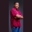Related Tags

swift

# How to get the total number of values present in a range in SwiftTheodore Kelechukwu Onyejiaku

### Overview

The total number of values present in a range can be found using the count method.

### Syntax

range.count


### Return value

The returned value is an integer and represents the total number of values present in a particular range.

### Code example

// create some ranges
let range1 = 1 ..< 6   // 1 to 5
let range2 = 10 ..< 50 // 10 to 49
let range3 = 0 ..< 0   // empty
let range4 = 3 ..< 3   // empty

// print the number of values
print(range1.count)  // 5
print(range2.count)  // 40
print(range3.count)  // 0
print(range4.count)  // 0
How to get the total number of a values in a Range in Swift

### Explanation

• Line 2–5: We create range variables.
• Line 8–11: The total number of values present in the range variables that we created were extracted using the count method. Finally, the results were printed to the console.

RELATED TAGS

swift

CONTRIBUTORTheodore Kelechukwu Onyejiaku
RELATED COURSES

View all Courses

Keep Exploring

Learn in-demand tech skills in half the time